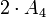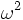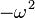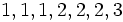# Linear representation theory of special linear group:SL(2,3)

Jump to: navigation, search
This article gives specific information, namely, linear representation theory, about a particular group, namely: special linear group:SL(2,3).
View linear representation theory of particular groups | View other specific information about special linear group:SL(2,3)

## Summary

Item Value
degrees of irreducible representations over a splitting field (such as$\mathbb{C}$ or$\overline{\mathbb{Q}}$) 1,1,1,2,2,2,3
grouped: 1 (3 times), 2 (3 times), 3 (1 time)
maximum: 3, lcm: 6, number: 7, sum of squares: 24, quasirandom degree: 1
Schur index values of irreducible representations over a splitting field 1,1,1,2,1,1,1
smallest ring of realization for all irreducible representations (characteristic zero)$\mathbb{Z}[e^{2\pi i/3}]$, same as$\mathbb{Z}[t]/(t^2 + t + 1)$
Same as ring generated by character values
minimal splitting field, i.e., smallest field of realization for all irreducible representations (characteristic zero)$\mathbb{Q}(e^{2\pi i/3}) = \mathbb{Q}[t]/(t^2 + t + 1) = \mathbb{Q}[\sqrt{-3}]$
Same as field generated by character values
condition for a field to be a splitting field characteristic not equal to 2 or 3 and must contain a primitive cube root of unity.
For a finite field of size$q$, this is equivalent to 6 dividing$q - 1$.
minimal splitting field in characteristic$p \ne 0,2,3$ Case$p \equiv 1 \pmod 6$: prime field$\mathbb{F}_p$
Case$p \equiv -1 \pmod 6$:$\mathbb{F}_{p^2}$, a quadratic extension of$\mathbb{F}_p$.
smallest size splitting field field:F7, i.e., the field of 7 elements.
degrees of irreducible representations over a non-splitting field (such as the field of rational numbers) 1,2,3,4,4
number: 5
orbits of irreducible representations over a splitting field under action of automorphism group 1 orbit of size 1 of degree 1 representations, 1 orbit of size 2 of degree 1 representations, 1 orbit of size 1 of degree 2 representations, 1 orbit of size 2 of degree 2 representations, 1 orbit of size 1 of degree 3 representations
number: 5
orbits of irreducible representations over a splitting field under action of Galois group Characteristic$p \equiv 1 \pmod 6$: all orbits of size 1.
Characteristic 0 or$p \equiv -1 \pmod 6$: 1 orbit of size 1 of degree 1 representations, 1 orbit of size 2 of degree 1 representations, 1 orbit of size 1 of degree 2 representations, 1 orbit of size 2 of degree 2 representations, 1 orbit of size 1 of degree 3 representations.
number: 5
orbits of irreducible representations over a splitting field under action of one-dimensional representations by multiplication, i.e., up to projective equivalence Orbit sizes: 3 (degree 1 representations), 3 (degree 2 representations), 1 (degree 3 representations)
number: 3

## Family contexts

Family name Parameter values General discussion of linear representation theory of family
double cover of alternating group degree$n = 4$, i.e., the group$2 \cdot A_4$ linear representation theory of double cover of alternating group
special linear group of degree two over a finite field of size$q$$q = 3$, i.e., field:F3, i.e., the group is$SL(2,3)$ linear representation theory of special linear group of degree two over a finite field
COMPARE AND CONTRAST: View linear representation theory of groups of order 24 to compare and contrast the linear representation theory with other groups of order 24.

## Irreducible representations

### Summary information

Below is summary information on irreducible representations that are absolutely irreducible, i.e., they remain irreducible in any bigger field, and in particular are irreducible in a splitting field. We assume that the characteristic of the field is not 2 or 3, except in the last two columns, where we consider what happens in characteristic 2 and characteristic 3.

Name of representation type Number of representations of this type Degree Schur index Criterion for field Kernel Quotient by kernel (on which it descends to a faithful representation) Characteristic 2 Characteristic 3
trivial 1 1 1 any whole group trivial group works works
one-dimensional nontrivial 2 1 1 must contain a primitive cube root of unity, or equivalently, the polynomial$t^2 + t + 1$ must split. Q8 in SL(2,3) (the 2-Sylow subgroup) cyclic group:Z3 works works, same as trivial
irreducible two-dimensional (quaternionic, rational character) 1 2 2  ? trivial subgroup, i.e., the representation is faithful special linear group:SL(2,3)  ?  ?
two-dimensional with non-rational character 2 2 1 must contain a primitive cube root of unity, or equivalently, the polynomial$t^2 + t + 1$ must split. trivial subgroup special linear group:SL(2,3)  ?  ?
three-dimensional 1 3 1 any center of special linear group:SL(2,3) alternating group:A4 indecomposable but not irreducible  ?

## Character table

FACTS TO CHECK AGAINST (for characters of irreducible linear representations over a splitting field):
Orthogonality relations: Character orthogonality theorem | Column orthogonality theorem
Separation results (basically says rows independent, columns independent): Splitting implies characters form a basis for space of class functions|Character determines representation in characteristic zero
Numerical facts: Characters are cyclotomic integers | Size-degree-weighted characters are algebraic integers
Character value facts: Irreducible character of degree greater than one takes value zero on some conjugacy class| Conjugacy class of more than average size has character value zero for some irreducible character | Zero-or-scalar lemma

In the table below, we denote by$\omega$ a primitive cube root of unity.

Representation/conjugacy class representative and size$\begin{pmatrix} 1 & 0 \\ 0 & 1 \\\end{pmatrix}$ (size 1)$\begin{pmatrix} -1 & 0 \\ 0 & -1 \\\end{pmatrix}$ (size 1)$\begin{pmatrix} 0 & -1 \\ 1 & 0 \\\end{pmatrix}$ (size 6)$\begin{pmatrix} 1 & 1 \\ 0 & 1 \\\end{pmatrix}$ (size 4)$\begin{pmatrix} 1 & -1 \\ 0 & 1 \\\end{pmatrix}$ (size 4)$\begin{pmatrix} -1 & 1 \\ 0 & -1 \\\end{pmatrix}$ (size 4)$\begin{pmatrix} -1 & -1 \\ 0 & -1 \\\end{pmatrix}$ (size 4)
trivial 1 1 1 1 1 1 1
nontrivial one-dimensional 1 1 1$\omega$$\omega^2$$\omega^2$$\omega$
nontrivial one-dimensional 1 1 1$\omega^2$$\omega$$\omega$$\omega^2$
irreducible two-dimensional (quaternionic, rational character) 2 -2 0 -1 -1 1 1
irreducible two-dimensional 2 -2 0$-\omega$$-\omega^2$$\omega^2$$\omega$
irreducible two-dimensional 2 -2 0$-\omega^2$$-\omega$$\omega$$\omega^2$
three-dimensional 3 3 -1 0 0 0 0

## Orthogonality relations and numerical checks

General statement Verification in this case
number of irreducible representations equals number of conjugacy classes Both numbers are equal to 7 (the degrees of irreducible representations are 1,1,1,2,2,2,3, and the sizes of conjugacy classes are 1,1,4,4,4,4,6). See also element structure of special linear group:SL(2,3).
As special linear group$SL(2,q)$,$q$ odd: Both numbers are equal to$q + 4 = 3 + 4 = 7$.
number of orbits of irreducible representations equals number of orbits under automorphism group Both numbers are 5. The orbit sizes for irreducible representations are: 1 (degree 1 representation), 2 (degree 1 representations), 1 (degree 2 representation), 2 (degree 2 representations), 1 (degree 3 representation). The orbit sizes for elements are 1,1,6,8 (2 conjugacy classes of size 4 each), 8 (2 conjugacy classes of size 4 each). See also element structure of special linear group:SL(2,3).
number of irreducible representations over rationals equals number of equivalence classes under rational conjugacy Both numbers are equal to 5. The irreducible representations over rationals have degrees 1, 2 (the sum of the two one-dimensional nontrivial representations), 3, 4 (double of the quaternionic representation), 4 (sum of the two other two-dimensional representations). The equivalence classes under rational conjugacy have sizes 1,1,6,8,8. See also element structure of special linear group:SL(2,3).
number of one-dimensional representations equals order of abelianization The derived subgroup is Q8 in SL(2,3), a 2-Sylow subgroup of order eight and index three. The abelianization is the corresponding quotient group, isomorphic to cyclic group:Z3, and has order three. The number of one-dimensional representations is also equal to three.
sum of squares of degrees of irreducible representations equals order of group The degrees of the irreducible representations are$1,1,1,2,2,2,3$. We have$1^2 + 1^2 + 1^2 + 2^2 + 2^2 + 2^2 + 3^2 = 24$.
degree of irreducible representation divides index of abelian normal subgroup The center (see center of special linear group:SL(2,3) is the unique nontrivial abelian normal subgroup, and it is a subgroup of order 2 and index 12. All the degrees of irreducible representations divide 12.
order of inner automorphism group bounds square of degree of irreducible representation The center of special linear group:SL(2,3) has order 2 and index 12, and the quotient group, the inner automorphism group, has order 12 (the quotient is$PSL(2,3)$ and is isomorphic to alternating group:A4). The squares of the degrees of irreducible representations are all less than or equal to this number (the largest square being$3^2 = 9$).
degree of irreducible representation is bounded by index of abelian subgroup There is an abelian 2-subnormal subgroup of order four and index six, isomorphic to cyclic group:Z4 (in fact, there is a conjugacy class of three such subgroups). All the degrees of irreducible representations are less than or equal to$6$.

## Isoclinism and projective representations

Please compare this with projective representation theory of alternating group:A4.

### Grouping by restriction to center

Restriction to center as a representation of center of special linear group:SL(2,3) which is isomorphic to cyclic group:Z2 (this determines, essentially, the cohomology class of the projective representation for the inner automorphism group) List of irreducible projective representations of the inner automorphism group (which is alternating group:A4) List of corresponding linear representations of special linear group:SL(2,3) List of degrees Sum of squares of degrees (should equal order of inner automorphism group, which is 12)
trivial representation trivial representation all the three one-dimensional representations, and the three-dimensional irreducible representation (which descends to the standard representation for alternating group:A4) 1,1,1,3 12
sign representation nontrivial two-dimensional projective representation all the two-dimensional representations 2,2,2 12

### Grouping by projective representation

Irreducible projective representation of inner automorphism group (which is alternating group:A4) Degree Size of stabilizer under action of one-dimensional representations of special linear group:SL(2,3) (or equivalently, of its abelianization, cyclic group:Z3) Size of orbit (equals order of abelianization (3) divided by size of stabilizer) List of irreducible representations of special linear group:SL(2,3)
trivial representation 1 1 3 trivial representation and the two other one-dimensional representations
nonlinear projective two-dimensional representation 2 1 3 all the three two-dimensional representations
linear three-dimensional representation (standard representation of alternating group:A4) 3 3 1 the unique three-dimensional representation

## GAP implementation

### Degrees of irreducible representations

The degrees of irreducible representations can be computed using the CharacterDegrees function:

gap> CharacterDegrees(SL(2,3));
[ [ 1, 3 ], [ 2, 3 ], [ 3, 1 ] ]

This says that there are three irreducible representations of degree one, three irreducible representations of degree two, and one irreducible representation of degree three.

### Character table

The characters of irreducible representations can be computed using Irr and CharacterTable as follows:

gap> Irr(CharacterTable(SL(2,3)));
[ Character( CharacterTable( SL(2,3) ), [ 1, 1, 1, 1, 1, 1, 1 ] ),
Character( CharacterTable( SL(2,3) ), [ 1, E(3)^2, E(3), 1, E(3), E(3)^2, 1
] ), Character( CharacterTable( SL(2,3) ),
[ 1, E(3), E(3)^2, 1, E(3)^2, E(3), 1 ] ),
Character( CharacterTable( SL(2,3) ), [ 2, 1, 1, -2, -1, -1, 0 ] ),
Character( CharacterTable( SL(2,3) ), [ 2, E(3), E(3)^2, -2, -E(3)^2,
-E(3), 0 ] ), Character( CharacterTable( SL(2,3) ),
[ 2, E(3)^2, E(3), -2, -E(3), -E(3)^2, 0 ] ),
Character( CharacterTable( SL(2,3) ), [ 3, 0, 0, 3, 0, 0, -1 ] ) ]

A nicer display of the character table can be achieved with the Display function:

gap> Display(CharacterTable(SL(2,3)));
CT1

2  3  1  1  3   1   1  2
3  1  1  1  1   1   1  .

1a 6a 6b 2a  3a  3b 4a

X.1     1  1  1  1   1   1  1
X.2     1  A /A  1  /A   A  1
X.3     1 /A  A  1   A  /A  1
X.4     2  1  1 -2  -1  -1  .
X.5     2 /A  A -2  -A -/A  .
X.6     2  A /A -2 -/A  -A  .
X.7     3  .  .  3   .   . -1

A = E(3)^2
= (-1-ER(-3))/2 = -1-b3

### Irreducible representations

The irreducible representations can be computed using IrreducibleRepresentations as follows:

gap> IrreducibleRepresentations(SL(2,3));
[ CompositionMapping( [ (4,5,6)(7,9,8), (2,7,3,4)(5,8,9,6) ] ->
[ [ [ 1 ] ], [ [ 1 ] ] ], <action isomorphism> ),
CompositionMapping( [ (4,5,6)(7,9,8), (2,7,3,4)(5,8,9,6) ] ->
[ [ [ E(3)^2 ] ], [ [ 1 ] ] ], <action isomorphism> ),
CompositionMapping( [ (4,5,6)(7,9,8), (2,7,3,4)(5,8,9,6) ] ->
[ [ [ E(3) ] ], [ [ 1 ] ] ], <action isomorphism> ),
CompositionMapping( [ (4,5,6)(7,9,8), (2,7,3,4)(5,8,9,6) ] ->
[ [ [ E(3), -E(3) ], [ 0, E(3)^2 ] ], [ [ E(3), 1 ], [ E(3), -E(3) ] ]
], <action isomorphism> ),
CompositionMapping( [ (4,5,6)(7,9,8), (2,7,3,4)(5,8,9,6) ] ->
[ [ [ E(3)^2, E(3) ], [ 0, 1 ] ],
[ [ -E(3)^2, -E(3) ], [ -E(3), E(3)^2 ] ] ], <action isomorphism> ),
CompositionMapping( [ (4,5,6)(7,9,8), (2,7,3,4)(5,8,9,6) ] ->
[ [ [ E(3), E(3)^2 ], [ 0, 1 ] ], [ [ 0, 1 ], [ -1, 0 ] ]
], <action isomorphism> ),
CompositionMapping( [ (4,5,6)(7,9,8), (2,7,3,4)(5,8,9,6) ] ->
[ [ [ 0, 0, 1 ], [ 0, 1, 0 ], [ -1, -1, -1 ] ],
[ [ 0, 1, 0 ], [ 1, 0, 0 ], [ -1, -1, -1 ] ] ], <action isomorphism> ) ]